# Kerala Board Class 10 Chemistry March 2019 Solved

This solved question paper is also a perfect tool for you to self-evaluate your exam preparation level. These Kerala Board SSLC previous year question paper designed based on the latest curriculum and syllabus will help you to prepare most competently for the board exams.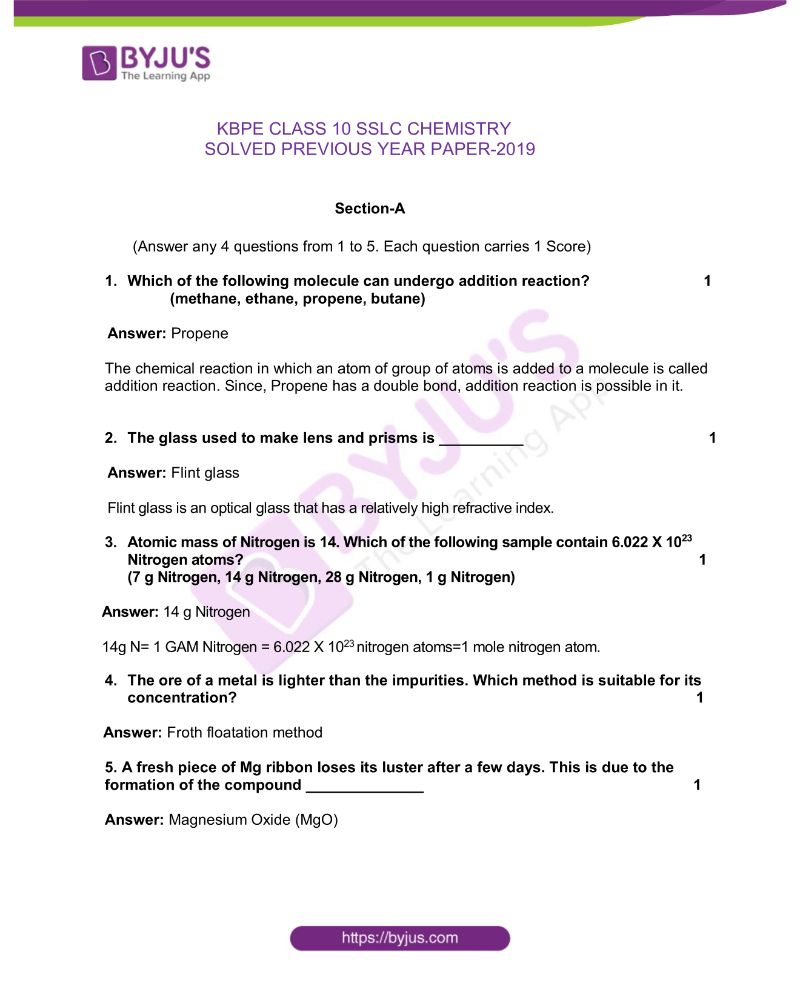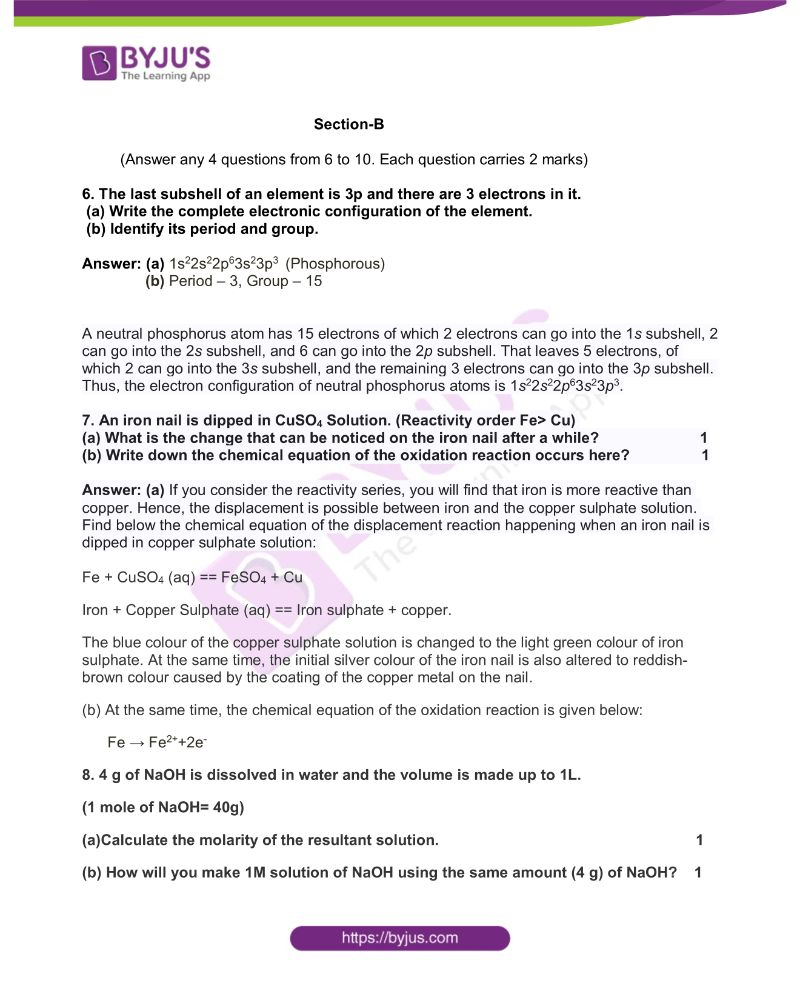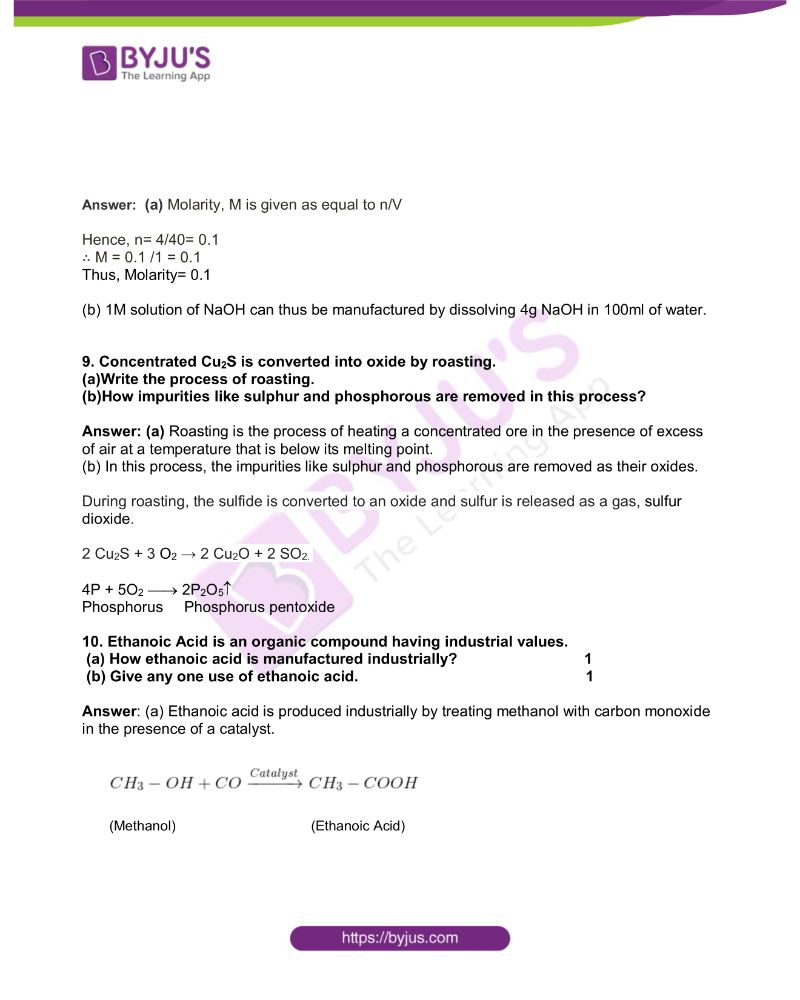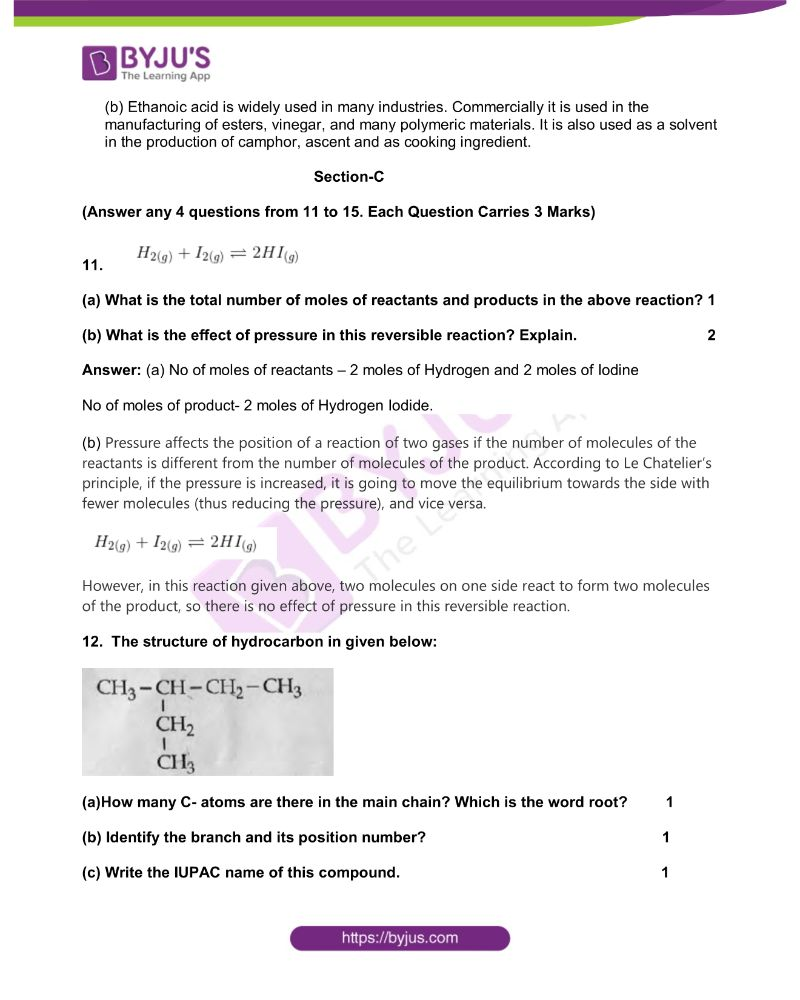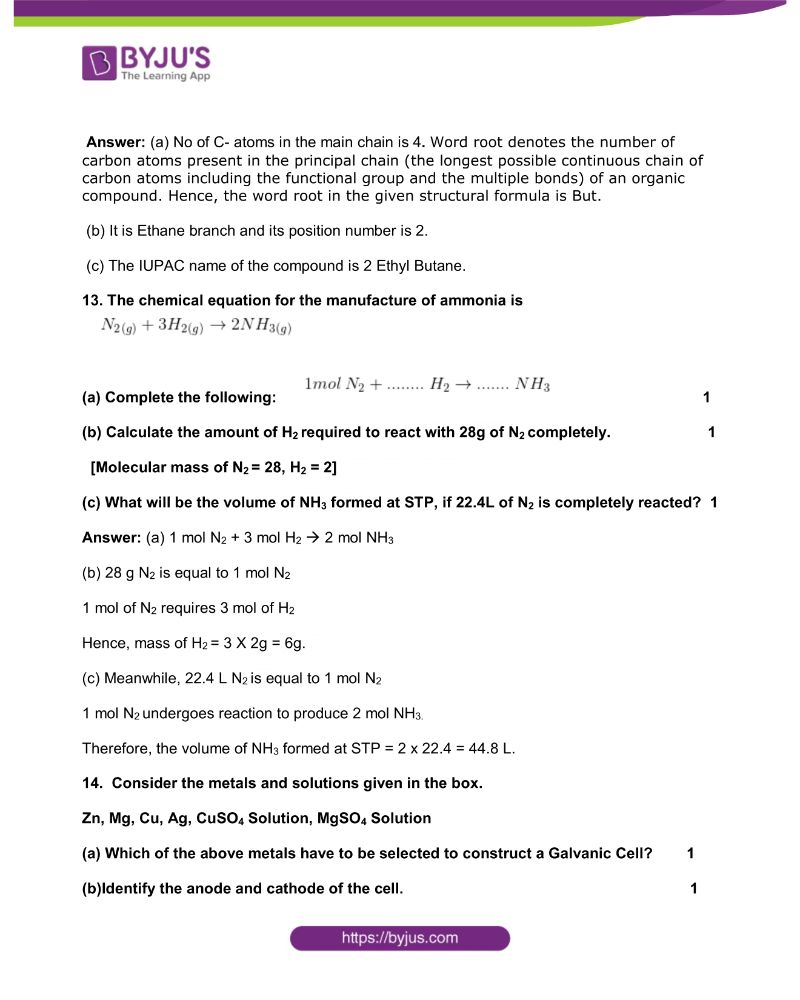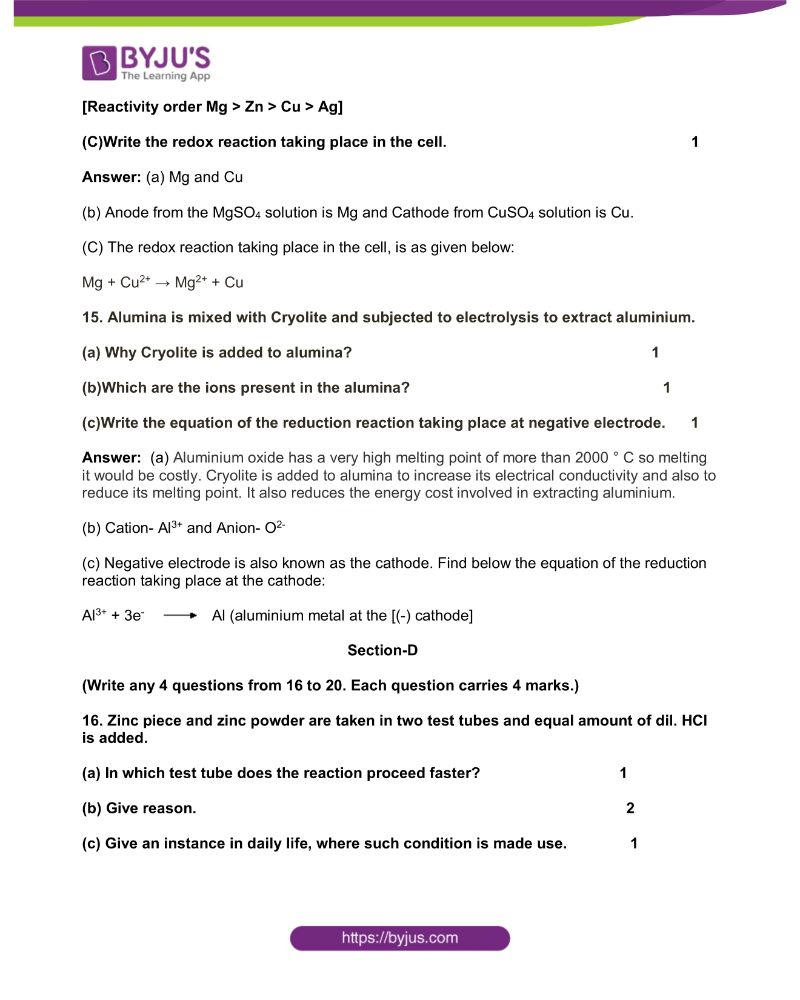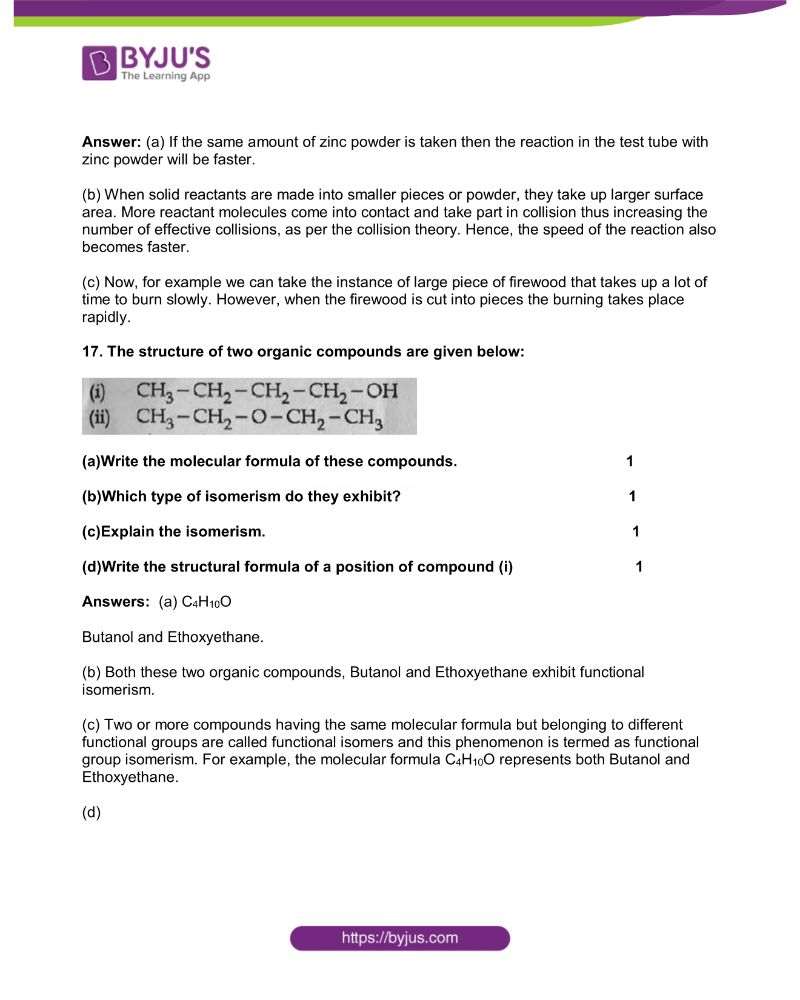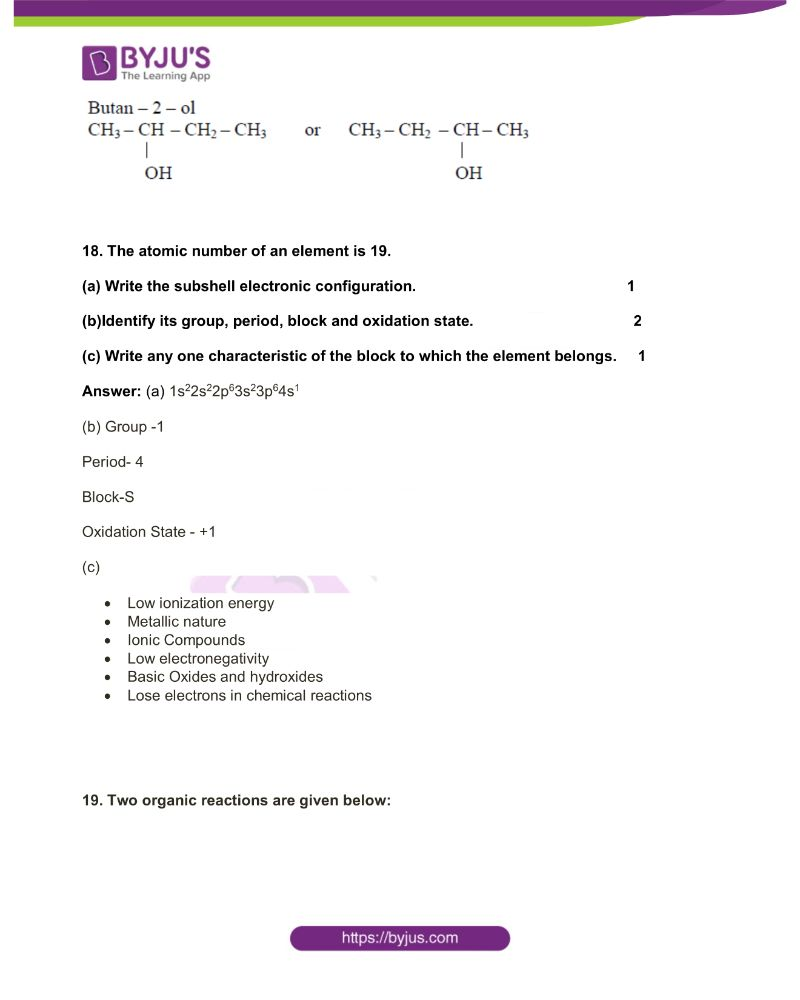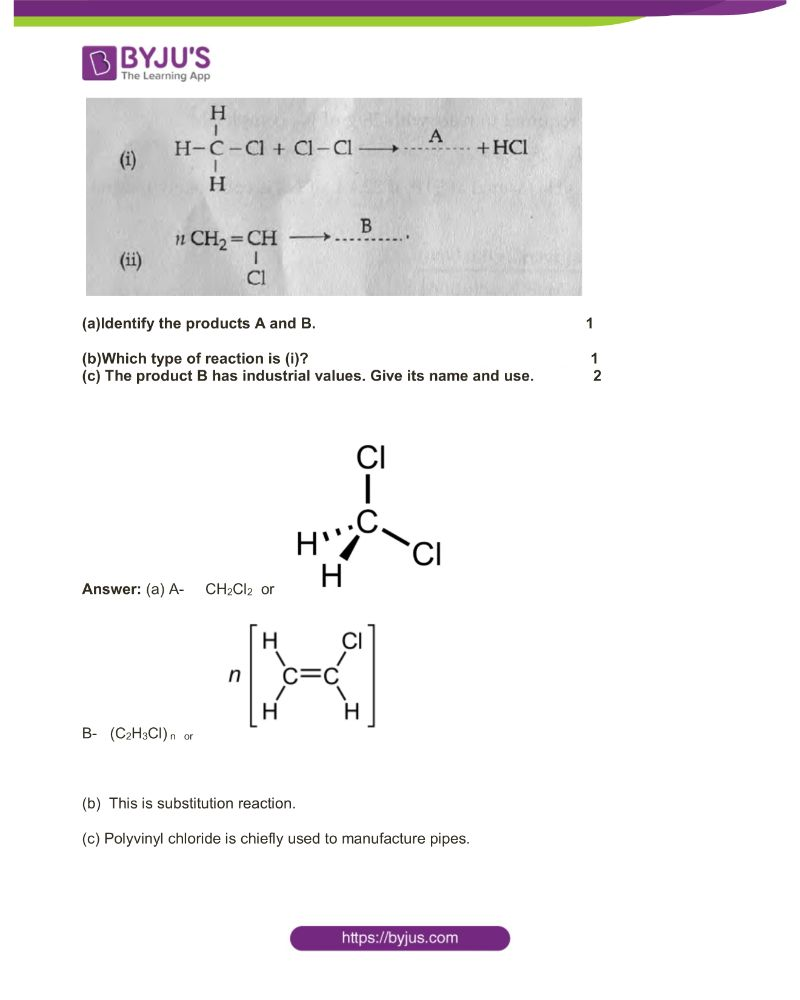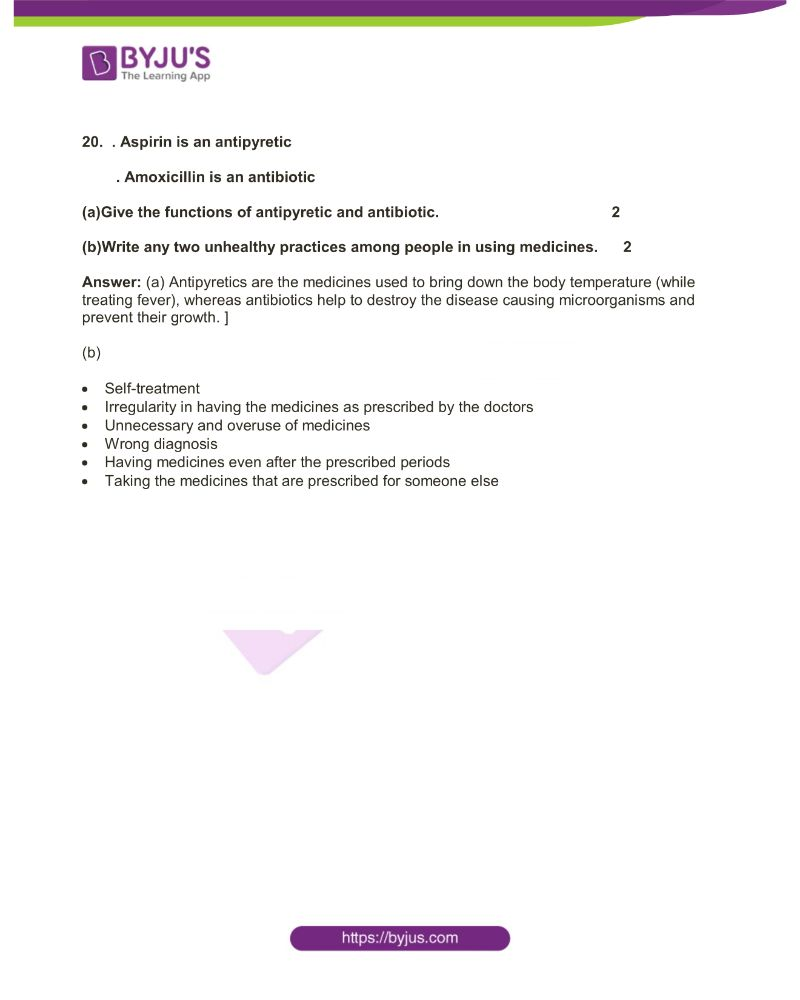## Kerala State Board SSLC Class 10 Chemistry Questions with Solutions

Section-A

(Answer any 4 questions from 1 to 5. Each question carries 1 Score)

1. Which of the following molecule can undergo addition reaction?

(methane, ethane, propene, butane)

The chemical reaction in which an atom of a group of atoms is added to a molecule is called addition reaction. Since Propene has a double bond, addition reaction is possible in it.

2. The glass used to make lens and prisms is __________

Flint glass is an optical glass that has a relatively high refractive index.

3. Atomic mass of Nitrogen is 14. Which of the following samples contain 6.022 X 1023 Nitrogen atoms?

(7 g Nitrogen, 14 g Nitrogen, 28 g Nitrogen, 1 g Nitrogen)

14g N= 1 GAM Nitrogen = 6.022 X 1023 nitrogen atoms=1 mole nitrogen atom.

4. The ore of the metal is lighter than the impurities. Which method is suitable for its concentration?

5. A fresh piece of Mg ribbon loses its lustre after a few days. This is due to the formation of the compound ______________

Section-B

(Answer any 4 questions from 6 to 10. Each question carries 2 marks)

6. The last subshell of an element is 3p and there are 3 electrons in it.

(a) Write the complete electronic configuration of the element.

(b) Identify its period and group.

(b) Period – 3, Group – 15

A neutral phosphorus atom has 15 electrons of which 2 electrons can go into the 1s subshell, 2 can go into the 2s subshell, and 6 can go into the 2p subshell. That leaves 5 electrons, of which 2 can go into the 3s subshell, and the remaining 3 electrons can go into the 3p subshell. Thus, the electron configuration of neutral phosphorus atoms is 1s22s22p63s23p3.

7. An iron nail is dipped in CuSO4 Solution. (Reactivity order Fe> Cu)

(a) What is the change that can be noticed on the iron nail after a while?

(b) Write down the chemical equation of the oxidation reaction occurs here?

Answer: (a) If you consider the reactivity series, you will find that iron is more reactive than copper. Hence, the displacement is possible between iron and the copper sulphate solution. Find below the chemical equation of the displacement reaction happening when an iron nail is dipped in copper sulphate solution:

Fe + CuSO4 (aq) == FeSO4 + Cu

Iron + Copper Sulphate (aq) == Iron sulphate + copper.

The blue colour of the copper sulphate solution is changed to the light green colour of iron sulphate. At the same time, the initial silver colour of the iron nail is also altered to reddish-brown colour caused by the coating of the copper metal on the nail.

(b) At the same time, the chemical equation of the oxidation reaction is given below:

Fe → Fe2++2e

8. 4 g of NaOH is dissolved in water and the volume is made up to 1L.

(1 mole of NaOH= 40g)

(a)Calculate the molarity of the resultant solution.

(b) How will you make 1M solution of NaOH using the same amount (4 g) of NaOH?

Answer: (a) Molarity, M is given as equal to n/V

Hence, n= 4/40= 0.1

∴ M = 0.1 /1 = 0.1

Thus, Molarity= 0.1

(b) 1M solution of NaOH can thus be manufactured by dissolving 4g NaOH in 100ml of water.

9. Concentrated Cu2S is converted into oxide by roasting.

(a)Write the process of roasting.

(b)How impurities like sulphur and phosphorus are removed in this process?

Answer: (a) Roasting is the process of heating a concentrated ore in the presence of excess air at a temperature that is below its melting point.

(b) In this process, the impurities like sulphur and phosphorus are removed as their oxides.

During roasting, the sulfide is converted to an oxide and sulfur is released as a gas, sulfur dioxide.

2 Cu2S + 3 O2 → 2 Cu2O + 2 SO2.

4P + 5O2 ⎯→ 2P2O5

Phosphorus Phosphorus pentoxide

10. Ethanoic Acid is an organic compound having industrial values.

(a) How ethanoic acid is manufactured industrially?

(b) Give anyone use of ethanoic acid.

Answer: (a) Ethanoic acid is produced industrially by treating methanol with carbon monoxide in the presence of a catalyst.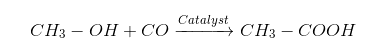(Methanol) (Ethanoic Acid)

(b) Ethanoic acid is widely used in many industries. Commercially it is used in the manufacturing of esters, vinegar, and many polymeric materials. It is also used as a solvent in the production of camphor, ascent and as a cooking ingredient.

Section-C

(Answer any 4 questions from 11 to 15. Each Question Carries 3 Marks)

11. H2(g) + I2(g) ⇋ 2HI(g)

(a) What is the total number of moles of reactants and products in the above reaction?

(b) What is the effect of pressure on this reversible reaction? Explain.

Answer: (a) No of moles of reactants – 2 moles of Hydrogen and 2 moles of Iodine

No of moles of product- 2 moles of Hydrogen Iodide.

(b) Pressure affects the position of a reaction of two gases if the number of molecules of the reactants is different from the number of molecules of the product. According to Le Chatelier’s principle, if the pressure is increased, it is going to move the equilibrium towards the side with fewer molecules (thus reducing the pressure), and vice versa.

H2(g) + I2(g) ⇋ 2HI(g)

However, in this reaction given above, two molecules on one side react to form two molecules of the product, so there is no effect of pressure in this reversible reaction.

12. The structure of hydrocarbon in given below: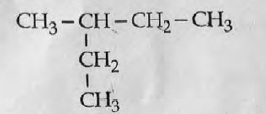(a)How many C- atoms are there in the main chain? Which is the word root? 1

(b) Identify the branch and its position number?

(c) Write the IUPAC name of this compound.

Answer: (a) No of C- atoms in the main chain is 4. Word root denotes the number of carbon atoms present in the principal or main chain (the longest possible continuous chain of carbon atoms, including the functional group and the multiple bonds) of an organic compound. Hence, the word root in the given structural formula is But.

(b) It is Ethane branch and its position number is 2.

(c) The IUPAC name of the compound is 2 Ethyl Butane.

13. The chemical equation for the manufacture of ammonia is N2(g) + 3H2(g) → 2 NH 3(g)

(a) Complete the following: 1mol N2 + ….. H2 → NH3

(b) Calculate the amount of H2 required to react with 28g of N2 completely.

[Molecular mass of N2 = 28, H2 = 2]

(c) What will be the volume of NH3 formed at STP, if 22.4L of N2 is completely reacted?

Answer: (a) 1 mol N2 + 3 mol H2 🡪 2 mol NH3

(b) 28 g N2 is equal to 1 mol N2

1 mol of N2 requires 3 mol of H2

Hence, mass of H2 = 3 X 2g = 6g.

(c) Meanwhile, 22.4 L N2 is equal to 1 mol N2

1 mol N2 undergoes a reaction to produce 2 mol NH3.

Therefore, the volume of NH3 formed at STP = 2 x 22.4 = 44.8 L.

14. Consider the metals and solutions given in the box.

Zn, Mg, Cu, Ag, CuSO4 Solution, MgSO4 Solution

(a) Which of the above metals have to be selected to construct a Galvanic Cell?

(b)Identify the anode and cathode of the cell.

[Reactivity order Mg > Zn > Cu > Ag]

(C)Write the redox reaction taking place in the cell.

(b) Anode from the MgSO4 solution is Mg and Cathode from CuSO4 solution is Cu.

(C) The redox reaction taking place in the cell is as given below:

Mg + Cu2+ → Mg2+ + Cu

15. Alumina is mixed with Cryolite and subjected to electrolysis to extract aluminium.

(a) Why Cryolite is added to alumina?

(b)Which are the ions present in the alumina?

(c)Write the equation of the reduction reaction taking place at the negative electrode.

Answer: (a) Aluminium oxide has a very high melting point of more than 2000 ° C so melting it would be costly. Cryolite is added to alumina to increase its electrical conductivity and also to reduce its melting point. It also reduces the energy cost involved in extracting aluminium.

(b) Cation- Al3+ and Anion- O2-

(c) Negative electrode is also known as the cathode. Find below the equation of the reduction reaction taking place at the cathode:

Al3+ + 3e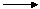Al (aluminium metal at the [(-) cathode]

Section-D

(Write any 4 questions from 16 to 20. Each question carries 4 marks.)

16. Zinc piece and zinc powder are taken in two test tubes and an equal amount of dil. HCl is added.

(a) In which test tube does the reaction proceed faster?

(b) Give reason.

(c) Give an instance in daily life, where such condition is made use.

Answer: (a) If the same amount of zinc powder is taken then the reaction in the test tube with zinc powder will be faster.

(b) When solid reactants are made into smaller pieces or powder, they take up a larger surface area. More reactant molecules come into contact and take part in collision thus increasing the number of effective collisions, as per the collision theory. Hence, the speed of the reaction also becomes faster.

(c) Now, for example, we can take the instance of a large piece of firewood that takes up a lot of time to burn slowly. However, when the firewood is cut into pieces the burning takes place rapidly.

17. The structure of two organic compounds are given below:

(i) CH3 – CH2 – CH2 – CH2 – OH

(ii) CH3 -CH2 – O – CH2 – CH3

(a)Write the molecular formula of these compounds.

(b)Which type of isomerism do they exhibit?

(c)Explain isomerism.

(d)Write the structural formula of a position of compound (i)

Butanol and Ethoxyethane.

(b) Both these two organic compounds, Butanol and Ethoxyethane exhibit functional isomerism.

(c) Two or more compounds having the same molecular formula but belonging to different functional groups are called functional isomers and this phenomenon is termed as functional group isomerism. For example, the molecular formula C4H10O represents both Butanol and Ethoxyethane.

(d)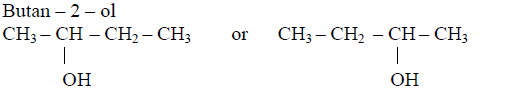18. The atomic number of an element is 19.

(a) Write the subshell electronic configuration.

(b)Identify its group, period, block and oxidation state.

(c) Write any one characteristic of the block to which the element belongs.

(b) Group -1

Period- 4

Block-S

Oxidation State – +1

(c)

• Low ionization energy
• Metallic nature
• Ionic Compounds
• Low electronegativity
• Basic Oxides and hydroxides
• Lose electrons in chemical reactions

19. Two organic reactions are given below: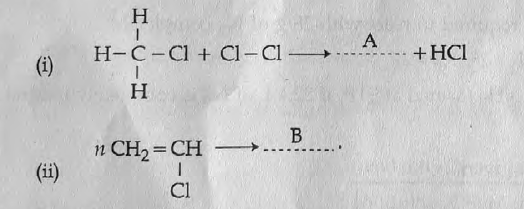(a)Identify the products A and B.

(b)Which type of reaction is (i)?
(c) The product B has industrial values. Give its name and use.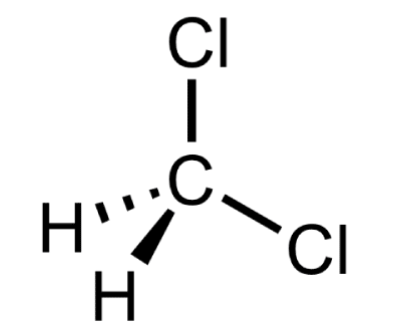B- (C2H3Cl) n or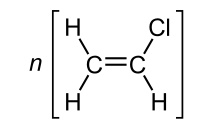(b) This is a substitution reaction.

(c) Polyvinyl chloride is chiefly used to manufacture pipes.

20. . Aspirin is an antipyretic

. Amoxicillin is an antibiotic

(a)Give the functions of antipyretic and antibiotic.

(b)Write any two unhealthy practices among people in using medicines.

Answer: (a) Antipyretics are the medicines used to bring down the body temperature (while treating fever), whereas antibiotics help to destroy the disease causing microorganisms and prevent their growth.

(b)

• Self-treatment
• Irregularity in having the medicines as prescribed by the doctors
• Unnecessary and overuse of medicines
• Wrong diagnosis
• Having medicines even after the prescribed periods
• Taking the medicines that are prescribed for someone else